Скачать презентацию IELM 231 IT for Logistics and Manufacturing Course

7f5ff030931615001b7445f3b13b64cc.ppt

• Количество слайдов: 22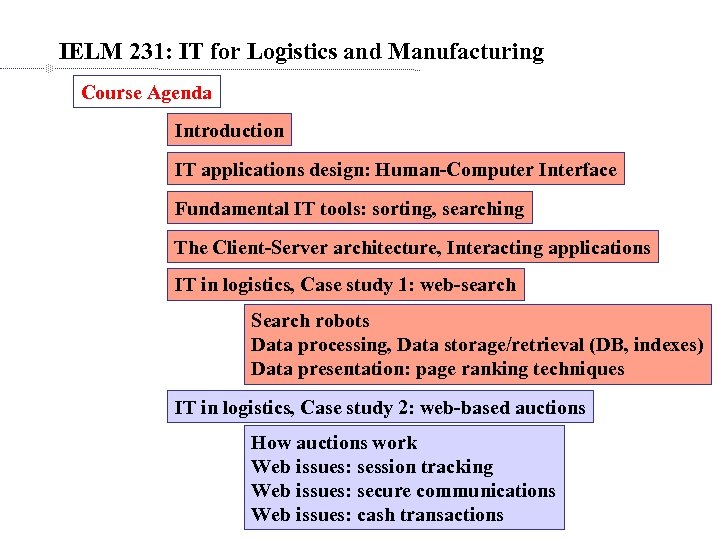IELM 231: IT for Logistics and Manufacturing Course Agenda Introduction IT applications design: Human-Computer Interface Fundamental IT tools: sorting, searching The Client-Server architecture, Interacting applications IT in logistics, Case study 1: web-search Search robots Data processing, Data storage/retrieval (DB, indexes) Data presentation: page ranking techniques IT in logistics, Case study 2: web-based auctions How auctions work Web issues: session tracking Web issues: secure communications Web issues: cash transactions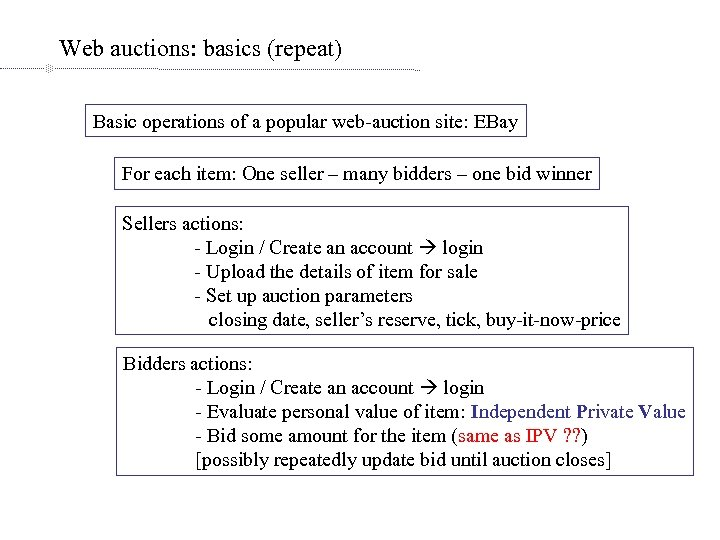Web auctions: basics (repeat) Basic operations of a popular web-auction site: EBay For each item: One seller – many bidders – one bid winner Sellers actions: - Login / Create an account login - Upload the details of item for sale - Set up auction parameters closing date, seller’s reserve, tick, buy-it-now-price Bidders actions: - Login / Create an account login - Evaluate personal value of item: Independent Private Value - Bid some amount for the item (same as IPV ? ? ) [possibly repeatedly update bid until auction closes]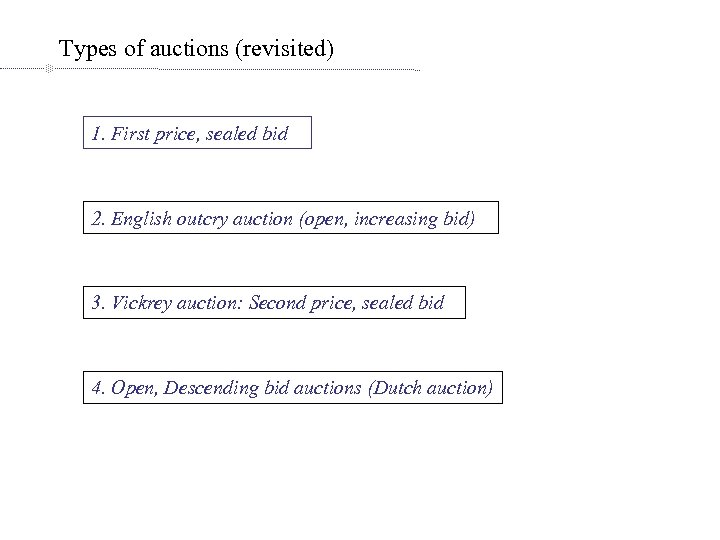Types of auctions (revisited) 1. First price, sealed bid 2. English outcry auction (open, increasing bid) 3. Vickrey auction: Second price, sealed bid 4. Open, Descending bid auctions (Dutch auction)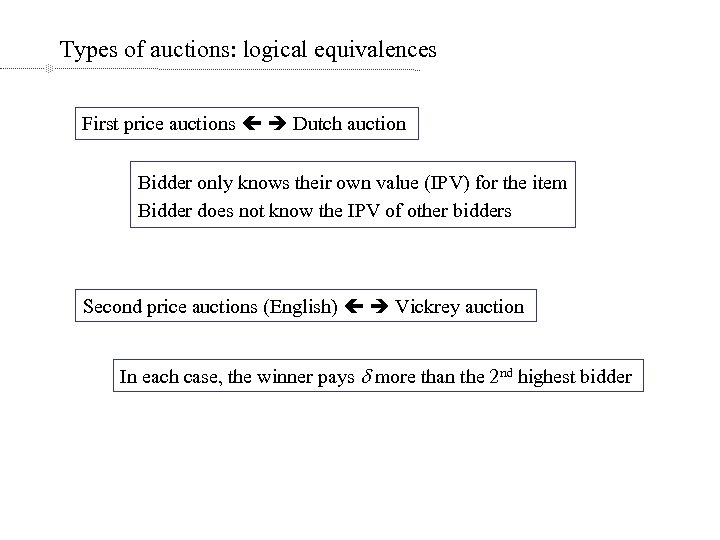Types of auctions: logical equivalences First price auctions Dutch auction Bidder only knows their own value (IPV) for the item Bidder does not know the IPV of other bidders Second price auctions (English) Vickrey auction In each case, the winner pays d more than the 2 nd highest bidder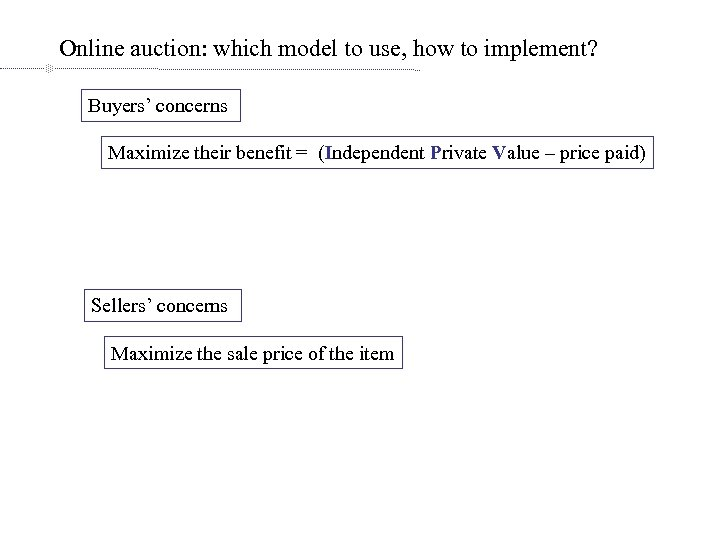Online auction: which model to use, how to implement? Buyers’ concerns Maximize their benefit = (Independent Private Value – price paid) Sellers’ concerns Maximize the sale price of the item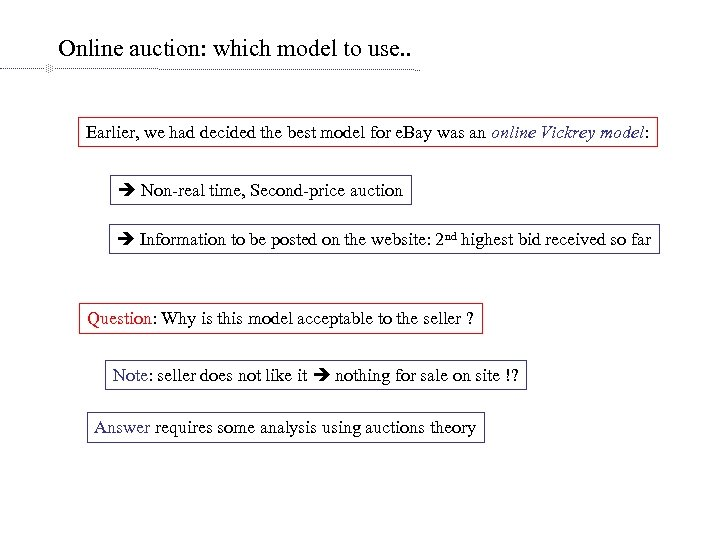Online auction: which model to use. . Earlier, we had decided the best model for e. Bay was an online Vickrey model: Non-real time, Second-price auction Information to be posted on the website: 2 nd highest bid received so far Question: Why is this model acceptable to the seller ? Note: seller does not like it nothing for sale on site !? Answer requires some analysis using auctions theory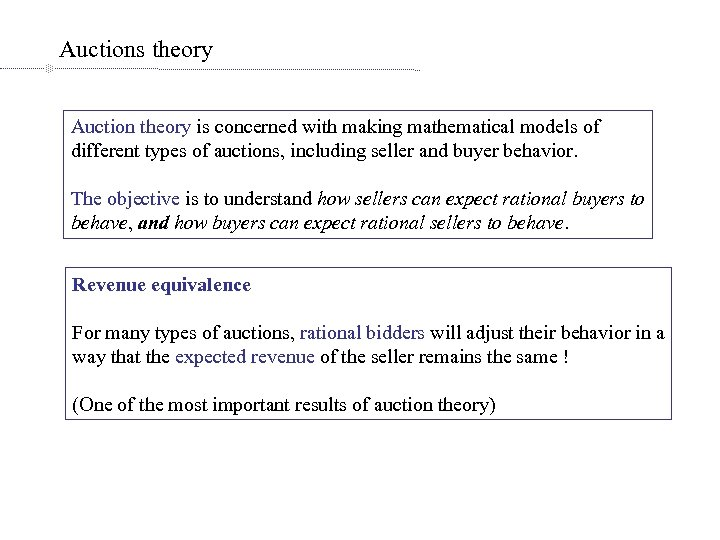Auctions theory Auction theory is concerned with making mathematical models of different types of auctions, including seller and buyer behavior. The objective is to understand how sellers can expect rational buyers to behave, and how buyers can expect rational sellers to behave. Revenue equivalence For many types of auctions, rational bidders will adjust their behavior in a way that the expected revenue of the seller remains the same ! (One of the most important results of auction theory)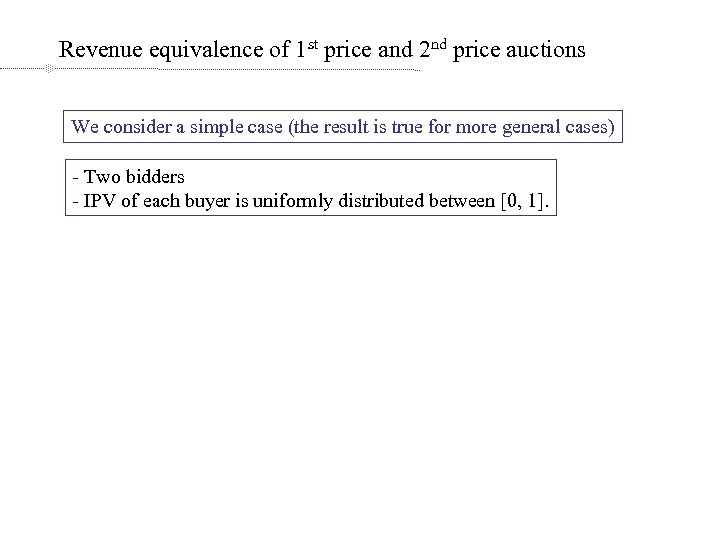Revenue equivalence of 1 st price and 2 nd price auctions We consider a simple case (the result is true for more general cases) - Two bidders - IPV of each buyer is uniformly distributed between [0, 1].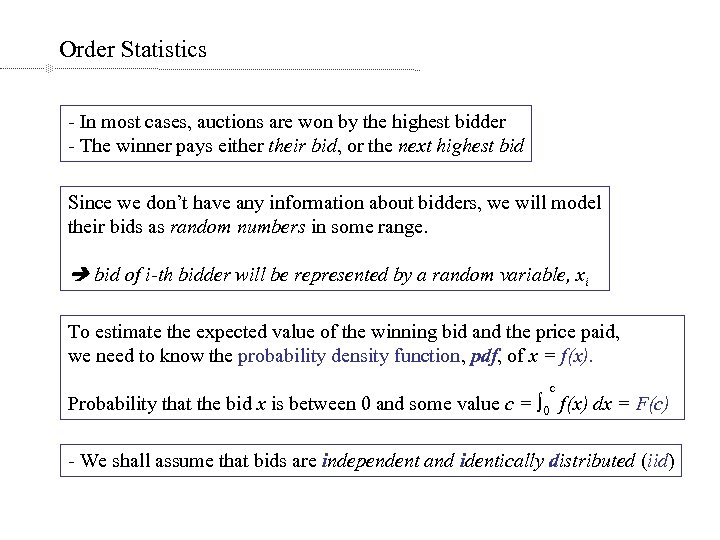Order Statistics - In most cases, auctions are won by the highest bidder - The winner pays either their bid, or the next highest bid Since we don’t have any information about bidders, we will model their bids as random numbers in some range. bid of i-th bidder will be represented by a random variable, xi To estimate the expected value of the winning bid and the price paid, we need to know the probability density function, pdf, of x = f(x). c Probability that the bid x is between 0 and some value c = ∫ 0 f(x) dx = F(c) - We shall assume that bids are independent and identically distributed (iid)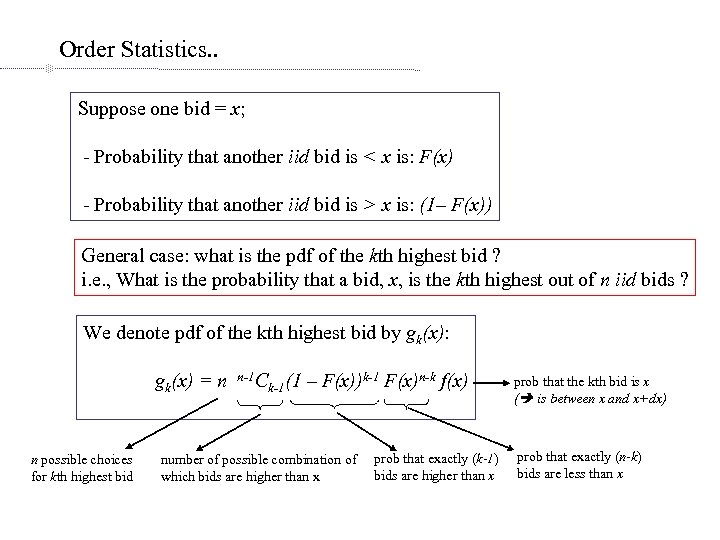Order Statistics. . Suppose one bid = x; - Probability that another iid bid is < x is: F(x) - Probability that another iid bid is > x is: (1– F(x)) General case: what is the pdf of the kth highest bid ? i. e. , What is the probability that a bid, x, is the kth highest out of n iid bids ? We denote pdf of the kth highest bid by gk(x): gk(x) = n n possible choices for kth highest bid n-1 C k-1(1 – F(x))k-1 F(x)n-k f(x) number of possible combination of which bids are higher than x prob that exactly (k-1) bids are higher than x prob that the kth bid is x ( is between x and x+dx) prob that exactly (n-k) bids are less than x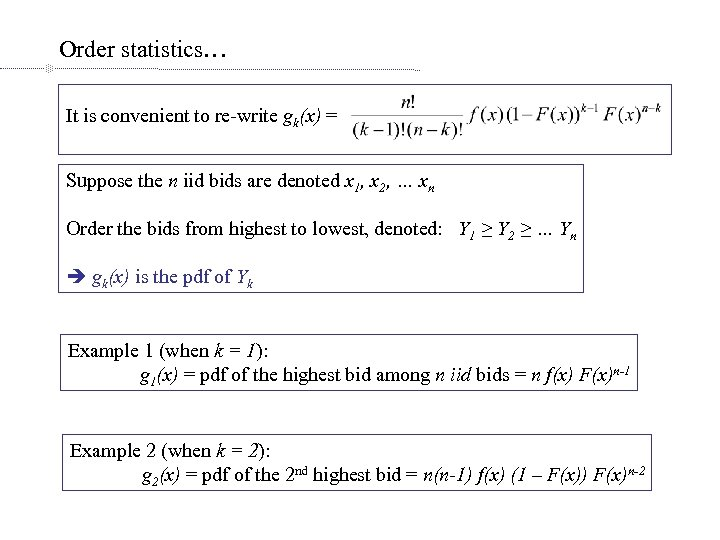Order statistics… It is convenient to re-write gk(x) = Suppose the n iid bids are denoted x 1, x 2, … xn Order the bids from highest to lowest, denoted: Y 1 ≥ Y 2 ≥ … Yn gk(x) is the pdf of Yk Example 1 (when k = 1): g 1(x) = pdf of the highest bid among n iid bids = n f(x) F(x)n-1 Example 2 (when k = 2): g 2(x) = pdf of the 2 nd highest bid = n(n-1) f(x) (1 – F(x)) F(x)n-2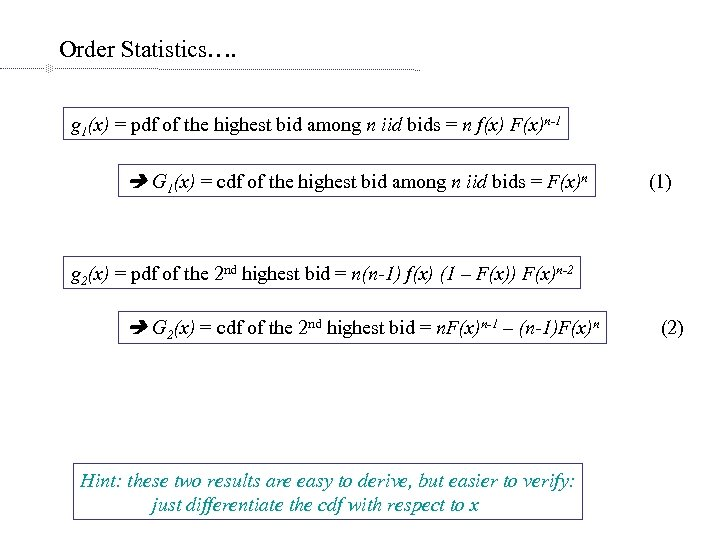Order Statistics…. g 1(x) = pdf of the highest bid among n iid bids = n f(x) F(x)n-1 G 1(x) = cdf of the highest bid among n iid bids = F(x)n (1) g 2(x) = pdf of the 2 nd highest bid = n(n-1) f(x) (1 – F(x)) F(x)n-2 G 2(x) = cdf of the 2 nd highest bid = n. F(x)n-1 – (n-1)F(x)n Hint: these two results are easy to derive, but easier to verify: just differentiate the cdf with respect to x (2)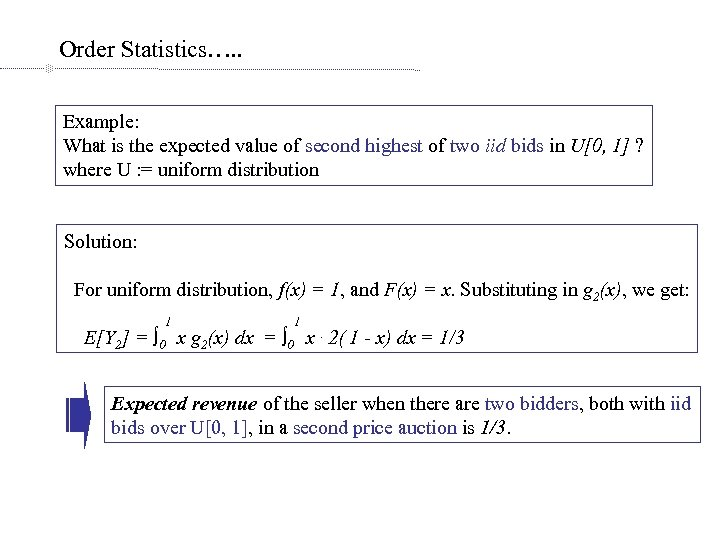Order Statistics…. . Example: What is the expected value of second highest of two iid bids in U[0, 1] ? where U : = uniform distribution Solution: For uniform distribution, f(x) = 1, and F(x) = x. Substituting in g 2(x), we get: 1 1 E[Y 2] = ∫ 0 x g 2(x) dx = ∫ 0 x. 2( 1 - x) dx = 1/3 Expected revenue of the seller when there are two bidders, both with iid bids over U[0, 1], in a second price auction is 1/3.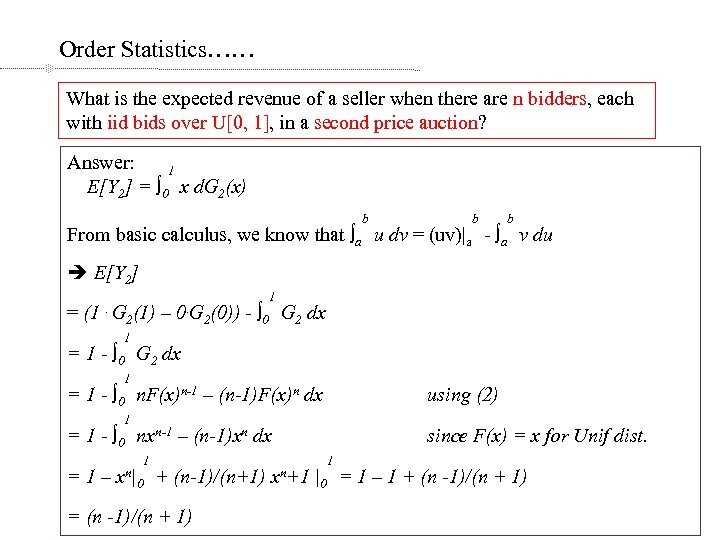Order Statistics…… What is the expected revenue of a seller when there are n bidders, each with iid bids over U[0, 1], in a second price auction? Answer: 1 E[Y 2] = ∫ 0 x d. G 2(x) b b b From basic calculus, we know that ∫a u dv = (uv)|a - ∫a v du E[Y 2] = (1 . G 2(1) 1 – 0. G 2(0)) 1 - ∫ 0 G 2 dx = 1 - ∫ 0 G 2 dx 1 = 1 - ∫ 0 n. F(x)n-1 – (n-1)F(x)n dx using (2) 1 = 1 - ∫ 0 nxn-1 – (n-1)xn dx =1– x n| 1 0 + (n-1)/(n+1) = (n -1)/(n + 1) xn+1 since F(x) = x for Unif dist. 1 |0 = 1 – 1 + (n -1)/(n + 1)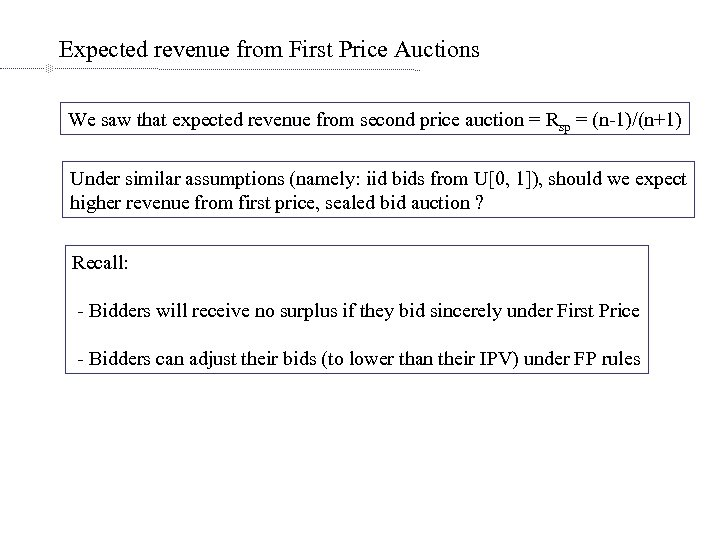Expected revenue from First Price Auctions We saw that expected revenue from second price auction = Rsp = (n-1)/(n+1) Under similar assumptions (namely: iid bids from U[0, 1]), should we expect higher revenue from first price, sealed bid auction ? Recall: - Bidders will receive no surplus if they bid sincerely under First Price - Bidders can adjust their bids (to lower than their IPV) under FP rules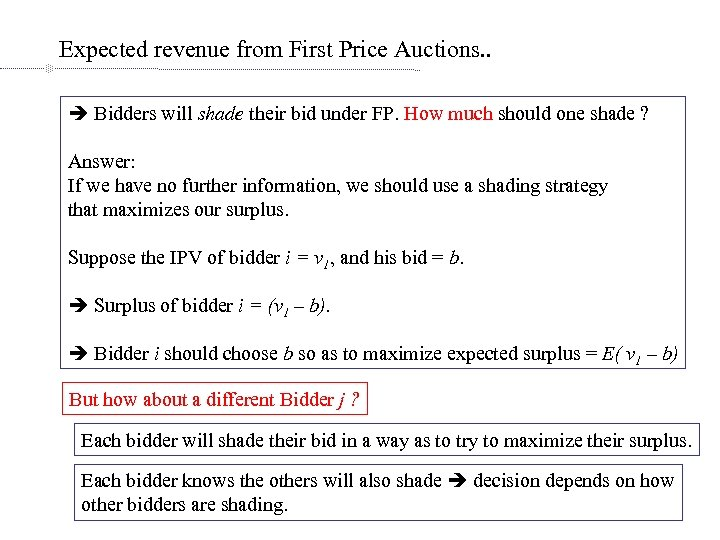Expected revenue from First Price Auctions. . Bidders will shade their bid under FP. How much should one shade ? Answer: If we have no further information, we should use a shading strategy that maximizes our surplus. Suppose the IPV of bidder i = v 1, and his bid = b. Surplus of bidder i = (v 1 – b). Bidder i should choose b so as to maximize expected surplus = E( v 1 – b) But how about a different Bidder j ? Each bidder will shade their bid in a way as to try to maximize their surplus. Each bidder knows the others will also shade decision depends on how other bidders are shading.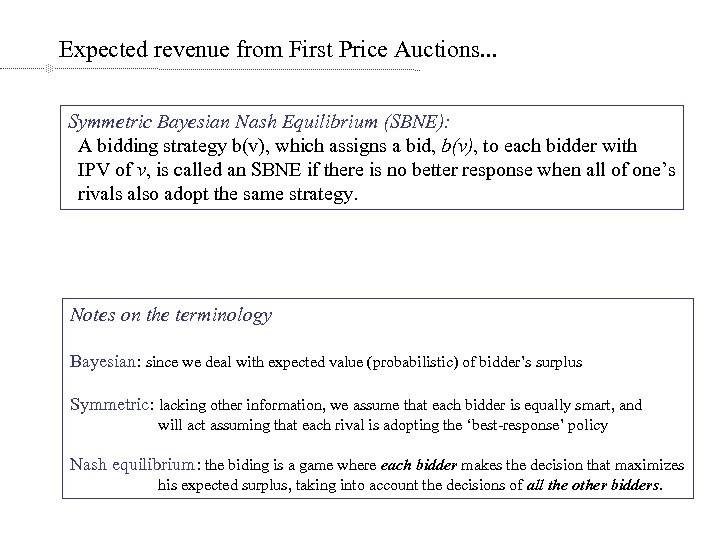Expected revenue from First Price Auctions. . . Symmetric Bayesian Nash Equilibrium (SBNE): A bidding strategy b(v), which assigns a bid, b(v), to each bidder with IPV of v, is called an SBNE if there is no better response when all of one’s rivals also adopt the same strategy. Notes on the terminology Bayesian: since we deal with expected value (probabilistic) of bidder’s surplus Symmetric: lacking other information, we assume that each bidder is equally smart, and will act assuming that each rival is adopting the ‘best-response’ policy Nash equilibrium: the biding is a game where each bidder makes the decision that maximizes his expected surplus, taking into account the decisions of all the other bidders.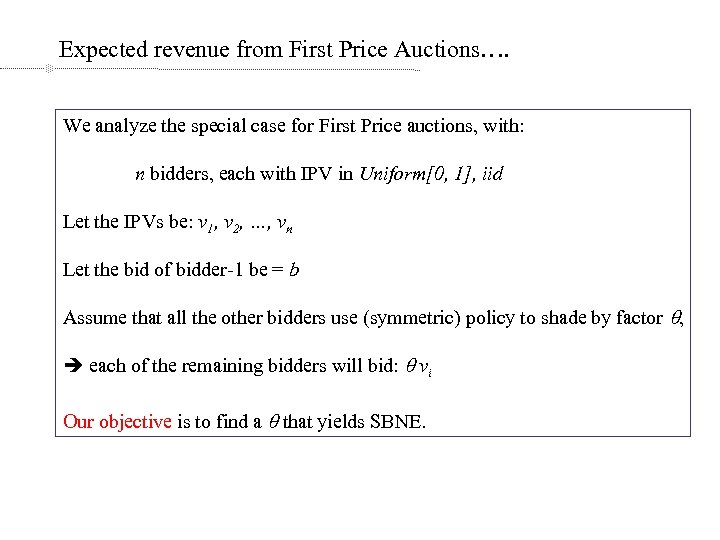Expected revenue from First Price Auctions…. We analyze the special case for First Price auctions, with: n bidders, each with IPV in Uniform[0, 1], iid Let the IPVs be: v 1, v 2, …, vn Let the bid of bidder-1 be = b Assume that all the other bidders use (symmetric) policy to shade by factor q, each of the remaining bidders will bid: q vi Our objective is to find a q that yields SBNE.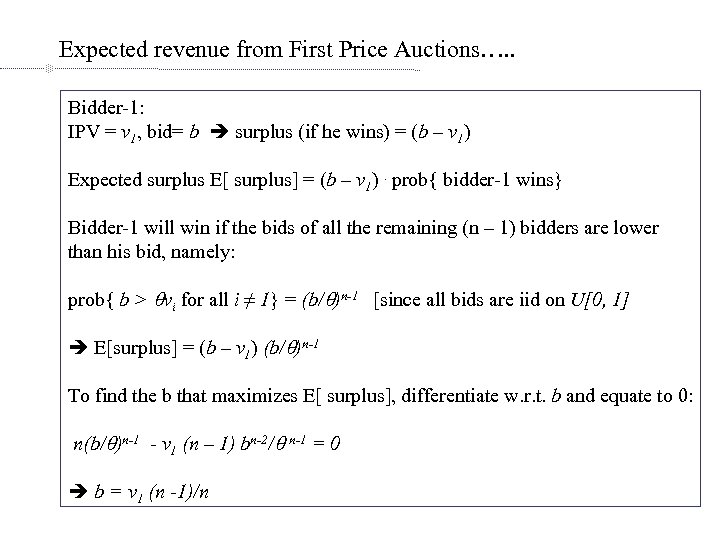Expected revenue from First Price Auctions…. . Bidder-1: IPV = v 1, bid= b surplus (if he wins) = (b – v 1) Expected surplus E[ surplus] = (b – v 1). prob{ bidder-1 wins} Bidder-1 will win if the bids of all the remaining (n – 1) bidders are lower than his bid, namely: prob{ b > qvi for all i ≠ 1} = (b/q)n-1 [since all bids are iid on U[0, 1] E[surplus] = (b – v 1) (b/q)n-1 To find the b that maximizes E[ surplus], differentiate w. r. t. b and equate to 0: n(b/q)n-1 - v 1 (n – 1) bn-2/q n-1 = 0 b = v 1 (n -1)/n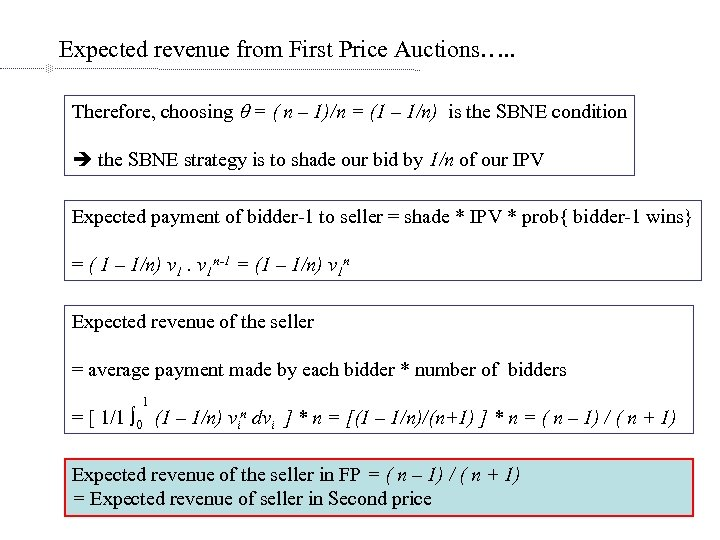Expected revenue from First Price Auctions…. . Therefore, choosing q = ( n – 1)/n = (1 – 1/n) is the SBNE condition the SBNE strategy is to shade our bid by 1/n of our IPV Expected payment of bidder-1 to seller = shade * IPV * prob{ bidder-1 wins} = ( 1 – 1/n) v 1 n-1 = (1 – 1/n) v 1 n Expected revenue of the seller = average payment made by each bidder * number of bidders 1 = [ 1/1 ∫ 0 (1 – 1/n) vin dvi ] * n = [(1 – 1/n)/(n+1) ] * n = ( n – 1) / ( n + 1) Expected revenue of the seller in FP = ( n – 1) / ( n + 1) = Expected revenue of seller in Second price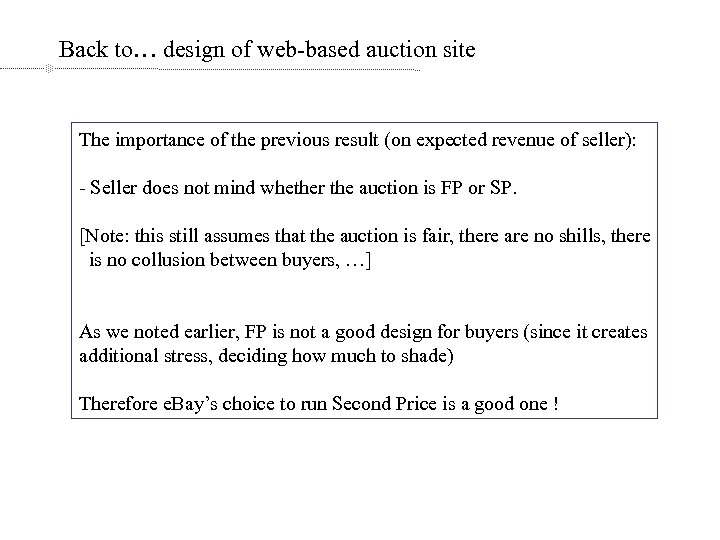Back to… design of web-based auction site The importance of the previous result (on expected revenue of seller): - Seller does not mind whether the auction is FP or SP. [Note: this still assumes that the auction is fair, there are no shills, there is no collusion between buyers, …] As we noted earlier, FP is not a good design for buyers (since it creates additional stress, deciding how much to shade) Therefore e. Bay’s choice to run Second Price is a good one !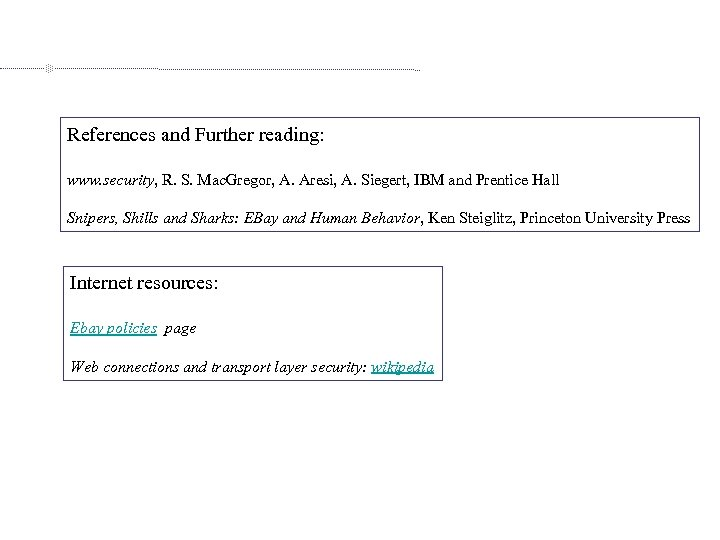References and Further reading: www. security, R. S. Mac. Gregor, A. Aresi, A. Siegert, IBM and Prentice Hall Snipers, Shills and Sharks: EBay and Human Behavior, Ken Steiglitz, Princeton University Press Internet resources: Ebay policies page Web connections and transport layer security: wikipedia